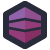Aaron was doing some work with a high-performance-computing platform. The kind of thing that handles complex numerical simulations divided across farms of CPUs, GPUs, FPGAs, and basically anything else that computes.

The system comes with a web GUI, and the code for the web GUI is… well… let's just look at the comment on a method.

``````/**
* This method executes a select query.
* @param filter associative array containing the table field as key and a value.
* @param table
* @param groupby array containing group by fields.
* @param orderby field to sort
* @param order ( desc or asc )
* @return A double array containing the query result.
*/
``````

Oh yeah, we're looking at PHP which generates SQL queries on the fly. And you know they happen via string concatenation. Even with that knowledge, however, it's actually probably worse than you think.

``````public function select( \$filter, \$table, \$groupby, \$orderby, \$order='desc', \$limit=0 )
{
\$filter_str = "";
\$groupby_str = "";
\$field_str = "";
\$is_first = TRUE;
\$join_annex = 0;
\$i = 0;
if ( \$table == MAIN_TABLE )
\$annex_schema = \$this->getSchema( ANNEX_TABLE );
else
\$annex_schema = null;

if( \$filter )
{
foreach( \$filter as \$field => \$value )
{
if ((\$annex_schema != null) && (in_array(\$field, array_keys(\$annex_schema))))
# need to join on annex table
\$join_annex = 1;

if( \$value != "")
{
\$filter_str .= (\$is_first ? '' : ' AND ');

if( preg_match( '`^.*(\*|\?).*\$`', \$value ) ) {
\$compar = ' LIKE ';
\$value = strtr( \$value, array( '?' => '.', '*' => '%' ));
} else {
\$compar = '=';
}

if ( \$field == PATH ) {
\$match_array = db_path_match(\$value);
if ( array_count_values( \$match_array ) == 1 )
\$filter_str .= \$field.\$compar.'\''.\$value.'\'';
else {
\$filter_str .= '(';
\$is_first_subexpr = TRUE;
foreach (\$match_array as \$expr ) {
if (preg_match( '`^.*(\%|\_).*\$`', \$expr ))
\$filter_str .= (\$is_first_subexpr ? '': ' OR ').\$field.' LIKE \''.\$expr.'\'';
else
\$filter_str .= (\$is_first_subexpr ? '': ' OR ').\$field.'=\''.\$expr.'\'';

\$is_first_subexpr = FALSE;
}
\$filter_str .= ')';
}
} else {
\$filter_str .= \$field.\$compar.'\''.\$value.'\'';
}
\$is_first = FALSE;
}
}
}

if( \$groupby )
{
\$is_first = TRUE;
foreach( \$groupby as \$field )
{
if ((\$annex_schema != null) && (in_array(\$field, array_keys(\$annex_schema))))
# need to join on annex table
\$join_annex = 1;

\$groupby_str = \$groupby_str.(\$is_first ? '' : ',').\$field;
\$is_first = FALSE;
}
}

/* /!\ is there a field in ANNEX_INFO? */

\$is_first = TRUE;
foreach( \$this->getSchema( \$table ) as \$field => \$type )
{
if( substr_count( \$type, "int" ) != 0 && \$groupby && \$field != "status" ) //TODO status case (no meaning here)
\$field_str .= ( \$is_first ? '' : ', ' )." SUM(".(\$join_annex ? "\$table." : "").\$field.")";
else
\$field_str .= ( \$is_first ? '' : ', ' ).(\$join_annex ? "\$table." : "").\$field;
\$is_first = FALSE;
}
if (\$join_annex) {
foreach( \$this->getSchema( ANNEX_TABLE ) as \$field => \$type )
{
if( substr_count( \$type, "int" ) != 0 && \$groupby && \$field != "status" ) //TODO status case (no meaning here)
\$field_str .= ( \$is_first ? '' : ', ' )." SUM(".(\$join_annex ? ANNEX_TABLE."." : "").\$field.")";
else
\$field_str .= ( \$is_first ? '' : ', ' ).(\$join_annex ? ANNEX_TABLE."." : "").\$field;
\$is_first = FALSE;
}
}

try
{
if (\$join_annex)
\$query = "SELECT ".\$field_str." FROM ".\$table." LEFT JOIN ".ANNEX_TABLE." ON \$table.id = ".ANNEX_TABLE.".id ".
( \$filter ? " WHERE ".\$filter_str : "" ).
( \$groupby ? " GROUP BY ".\$groupby_str : "" ).( \$orderby ? " ORDER BY ".\$orderby." ".\$order : "" ).
( \$limit > 0 ? " LIMIT \$limit" : "" );
else
\$query = "SELECT ".\$field_str." FROM ".\$table.( \$filter ? " WHERE ".\$filter_str : "" ).
( \$groupby ? " GROUP BY ".\$groupby_str : "" ).( \$orderby ? " ORDER BY ".\$orderby." ".\$order : "" ).
( \$limit > 0 ? " LIMIT \$limit" : "" );

\$result = \$this->connection->query( \$query );

\$i=0;
while( \$line = \$result->fetch( PDO::FETCH_ASSOC ) )
{
\$returned_result[\$i] = \$line;
\$i++;
if (\$i > MAX_SEARCH_RESULT)
{
echo "<b>ERROR: max result count exceeded</b><br>\n";
return null;
}
}
\$result->closeCursor();
\$this->rowNumber = \$i;

return \$returned_result;
}
catch( PDOException \$e )
{
echo 'Error: '.\$e->getMessage().'</br>';
}
}
``````

There's just so much in here. We start by detecting if we need to join to an `ANNEX_TABLE` based on whether or not our query target is the `MAIN_TABLE`. We loop over the filter, possibly joining to that table. We munge the comparison field with a regex, to see if we're doing an `=` comparison or a `LIKE` comparison.

The whole thing is a tortured pile of string munging and SQL injection and so much complexity to handle all of the weird little cases they've included.

The code is bad, through and through. But I think my favorite bits are the error handling: we just print an error to the page, complete with hard-coded `</br>` tags, which, um… the break tag is not meant to be an open/close tag pair. Even if we're doing older XHMTL style, the correct way to output that would be `<br />`.

Special credit to this line: `echo "<b>ERROR: max result count exceeded</b><br>\n";`, and that poor, useless `\n` hanging out at the end, too.[Advertisement] Continuously monitor your servers for configuration changes, and report when there's configuration drift. Get started with Otter today!